# SAT Math : How to find the length of the side of an equilateral triangle

## Example Questions

### Example Question #1 : How To Find The Length Of The Side Of An Equilateral Triangle

In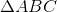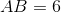and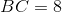. Which of the following CANNOT be the length of side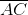Explanation:

The sum of the lengths of any two sides of a triangle must be greater than the length of third side.Therefore, because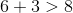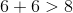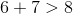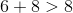and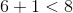, the correct answer must be.